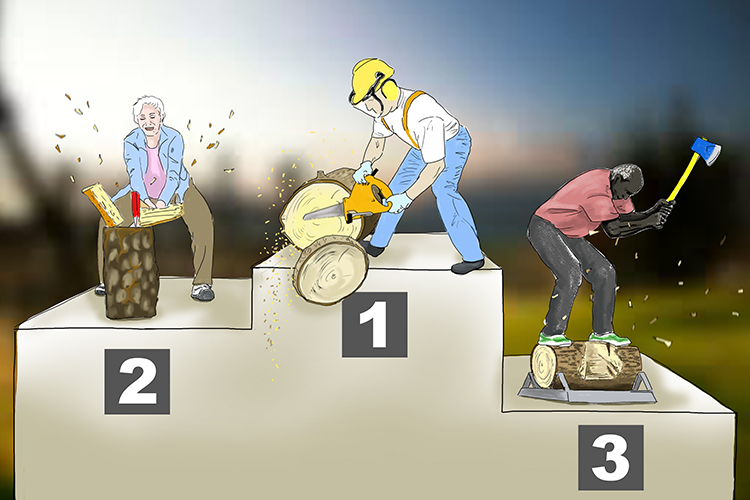# Reciprocal

Reciprocal = What to multiply a value by to get 1 (how to get ONE).At the log cutting competition, one person used a Reciprocating (reciprocal) saw and WON (1).

Example 1

What is the reciprocal of 5?

The reciprocal of 5 is worked out by putting a one under the 5 and turning it into a fraction and then turning the fraction upside down.

5 rArr5/1  turn over 1/5

So the reciprocal of 5 is  1/5 (or 0.2)

(To check multiply 0.2times5 and you get 1)

Example 2

What is the reciprocal of 8.

The reciprocal of 8 is worked out by putting a one under the 8 and turning it into a fraction and then turning the fraction upside down.

8rArr8/1  turn over 1/8

So the reciprocal of 8 is 1/8 (or 0.125)

(To check multiply 0.125times8 and you get 1)

Example 3

What is the reciprocal of 1000.

The reciprocal of 1000 is worked out by putting a one under the 1000 and turning it to a fraction and then turning the fraction upside down.

1000rArr1000/1  turn over  1/1000

So the reciprocal of 1000 is 1/1000 (or 0.001)

(To check multiply 0.001times1000 and you get 1)

Example 4

What is the reciprocal of 3/4

The reciprocal of 3/4 is worked out by putting a one under the 3/4 and turning it into a fraction and then turning the fraction upside down.

3/4rArr3/(4times1)  turn over (1times4)/3

So the reciprocal of 3/4 is 4/3

(To check multiply 3/4times4/3 and you get 1)